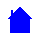## EL10A Course Outline

```Course Outline:
-----------------------
Lecture 1 -   historical perspective - unification theory, conservation principle and
the SI system of measurement as it relates to electrical quantities
Lecture 2 -   DC circuits - series and parallel circuits, resistance, voltage and current sources
Lecture 3 -   Kirchoff's laws with worked examples
Lecture 4 -   mesh current and node voltage analysis, planar circuits
Lecture 5 -   Cramers rule, determinants and Gaussian elimination
Lecture 6 -   Duality, Thevenin's and Norton's theorems and equivalent circuits
Lecture 7 -   superposition and maximum power transfer theorem
Lecture 8 -   storage components - capacitor and inductor
Lecture 9 -   transient analysis of first order circuits
Lecture 10 -  transient analysis of second order circuits
Lecture 11 -  AC circuits - behaviour of passive components, impedance, admittance, resonance and power
Lecture 12 -  class Test
Lecture 13 -  natural and forced response, series and parallel RLC circuits
Lecture 14 -  representation of sinusoids by phasor diagrams
Lecture 15 -  representation of sinusoids using complex numbers
Lecture 16 -  impedance, admittance, resonance and power using phasor diagrams or complex numbers
Lecture 17 -  AC steady state analysis
Lecture 18 -  mutual inductance, transformers
Lecture 19 -  steady state power analysis and polyphase circuits
Lecture 20 -  frequency domain analysis, bode plots
Lecture 21 -  operational amplifiers
Lecture 22 -  class Test
Lecture 23 -  op-amps - integrator and differentiator circuits
Lecture 24 -  op-amps - adder and subtractor circuits
Lecture 25 -  op-amps - multivibrator and waveform generator circuits
Lecture 26 -  power op-amps & revision

```EL10A Homepage